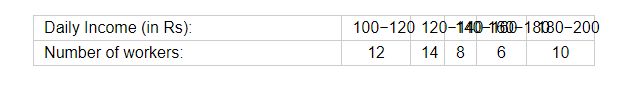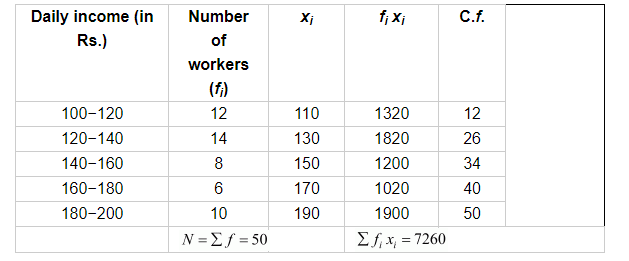# The following table gives the daily income of 50 workers of a factory:

Question:

The following table gives the daily income of 50 workers of a factory:Find the mean, mode and median of the above data.

Solution:

Consider the following table.Here, the maximum frequency is 14 so the modal class is 120−140.

Therefore,

$l=120$

$h=20$

$f=14$

$f_{1}=12$

$f_{2}=8$

$F=12$

Mean $=\frac{\sum f_{i} x_{i}}{\sum f}$

$=\frac{7260}{50}$

Mean $=145.20$

Thus, the mean daily income of the workers is Rs 145.20.

Median $=l+\frac{\frac{N}{2}-F}{f} \times h$

$=120+\frac{25-12}{14} \times 20$

$=120+\frac{13}{14} \times 20$

$=120+\frac{130}{7}$

Median $=138.57$

Thus, the median of the daily income of the workers is Rs 138.57.

Mode $=l+\frac{f-f_{1}}{2 f-f_{1}-f_{2}} \times h$

$=120+\frac{2}{8} \times 20$

$=120+5$

Mode $=125$

Thus, the mode of the daily income of the workers is Rs 125.